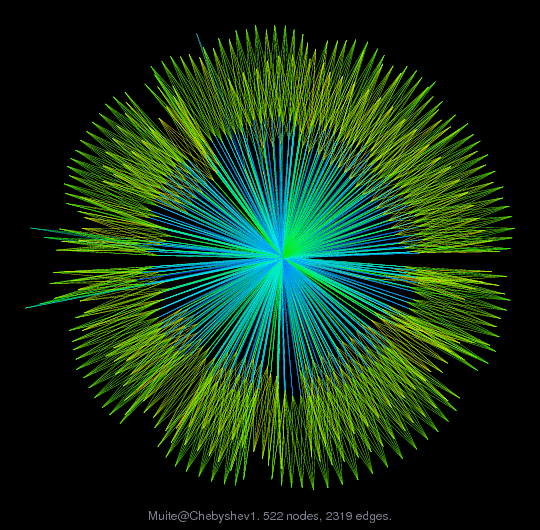Matrix: Muite/Chebyshev1

Description: Integration matrix, Chebyshev method, 4th order semilinear initial BVP(bipartite graph drawing) (graph drawing of A+A')• Matrix group: Muite
• download as a MATLAB mat-file, file size: 15 KB. Use UFget(1864) or UFget('Muite/Chebyshev1') in MATLAB.

 Matrix properties number of rows 261 number of columns 261 nonzeros 2,319 structural full rank? yes structural rank 261 # of blocks from dmperm 1 # strongly connected comp. 1 explicit zero entries 0 nonzero pattern symmetry 50% numeric value symmetry 0% type real structure unsymmetric Cholesky candidate? no positive definite? no

 author B. Muite editor T. Davis date 2007 kind structural problem 2D/3D problem? yes

Notes:

```Chebyshev integration matrix from Benson Muite, Oxford.  Details of the
matrices can be found in a preprint at http://www.maths.ox.ac.uk/~muite
entitled "A comparison of Chebyshev methods for solving fourth-order
semilinear initial boundary value problems," June 2007.   These matrices
are very ill-conditioned, partly because of the dense rows which are hard
to scale when coupled with the rest of the matrix.
```

 Ordering statistics: result nnz(chol(P*(A+A'+s*I)*P')) with AMD 1,803 Cholesky flop count 1.3e+04 nnz(L+U), no partial pivoting, with AMD 3,345 nnz(V) for QR, upper bound nnz(L) for LU, with COLAMD 1,040 nnz(R) for QR, upper bound nnz(U) for LU, with COLAMD 34,191

 SVD-based statistics: norm(A) 20277.2 min(svd(A)) 2.95871e-12 cond(A) 6.85338e+15 rank(A) 259 sprank(A)-rank(A) 2 null space dimension 2 full numerical rank? no singular value gap 4.61037e+06

 singular values (MAT file): click here SVD method used: s = svd (full (A)) ; status: ok